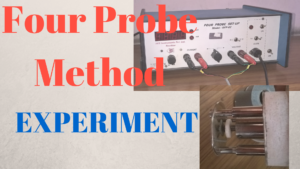# Four Probe Method – VIVA QUESTIONSThe following are some of the frequently asked questions for Viva of the Four Probe Experiment, to determine the resistivity and Energy Band gap of a semiconductor.

1. Why is Four-Probe method preferred over other conventional methods for measuring resistivity?
2. Why is the current kept constant for measuring the resistivity of a semiconductor using four probe at different temperatures?
A. In Four Probe Method, the voltage across the inner two probes at different temperatures is recorded. This voltage is an indication of resistance or resistivity only if V is proprtional to R or I is constant. This is why a constant current source is necessary.
3. How do the conductivities of metals and semiconductor depend on temperature?
A. The conductivity of a semiconductor increases with increasing temperature while that of a metal decreases with increasing temperature.
4. What is the equation giving the variation of conductivity/resistivity of a semiconductor with temperature?
A. Resistivity:$\rho=\rho_0 e^{E_g/2kT}$$\sigma=\sigma_0 e^{-E_g/2kT}$
5. For calculating the band gap from resistivity measurements why is it recommended to heat the sample over 100 degree Celsius?
6. What are the values of band gaps for Silicon and Germanium?
7. How does the band gap of a semiconductor vary with temperature?

Video:[wpedon id="7041" align="center"]

## 2 thoughts on “Four Probe Method – VIVA QUESTIONS”

1. Cant find all questons answers

2. 1. why the probes are pointed? or why do we use point contacts?
2. why do we use 4 probe setup, why not 2 probe setup?
3. why do we take readings during decreasing temperature?
4. why do we use spring contacts?